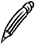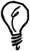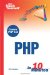# Arithmetic

As you would expect, PHP includes all the basic arithmetic operators. If you have not used another programming language, the symbols used might not all be obvious, so we'll quickly run through the basic rules of arithmetic in PHP.

#### Arithmetic Operators

Addition is performed with the plus symbol (+). This example adds 6 and 12 together and displays the result:

` echo 6 + 12; `

Subtraction is performed with the minus symbol (-), which is also used as a hyphen. This example subtracts 5 from 24:

` echo 24 - 5; `

The minus symbol can also be used to negate a number (for example, 20).

Multiplication is performed with the asterisk symbol (*). This example displays the product of 4 and 9:

` echo 4 * 9; `

Division is performed with the forward slash symbol (/). This example divides 48 by 12:

` echo 48 / 12; `Division When you divide two integers, the result is an integer if it divides exactly. Otherwise, it is a double. A fractional result is not rounded to an integer.

Modulus is performed by using the percent symbol (%). This example displays 3the remainder of 21 divided by 6:

` echo 21 % 6; `Modulus The modulus operator can be used to test whether a number is odd or even by using \$number % 2. The result will be 0 for all even numbers and 1 for all odd numbers (because any odd number divided by 2 has a remainder of 1).

#### Incrementing and Decrementing

In PHP you can increment or decrement a number by using a double plus (++) or double minus (--) symbol. The following statements both add one to \$number:

` \$number++; ++\$number; `

The operator can be placed on either side of a variable, and its position determines at what point the increment takes place.

This statement subtracts one from \$countdown before displaying the result:

` echo --\$countdown; `

However, the following statement displays the current value of \$countdown before decrementing it:

` echo \$countdown--; `

The increment and decrement operators are commonly used in loops. The following is a typical for loop, using a counter to repeat a section of code 10 times:

` for (\$count=1; \$count<=10; \$count++) {   echo "Count = \$count<br>"; } `

In this case, the code simply outputs the value of \$count for each pass of the loop.

#### Compound Operators

Compound operators provide a handy shortcut when you want to apply an arithmetic operation to an existing variable. The following example uses the compound addition operator to add six to the current value of \$count:

` \$count += 6; `

The effect of this is to take the initial value of \$count, add six to it, and then assign it back to \$count. In fact, the operation is equivalent to doing the following:

` \$count = \$count + 6; `

All the basic arithmetic operators have corresponding compound operators, as shown in Table 5.1.

Operator

Equivalent To

\$a += \$b

\$a = \$a + \$b;

\$a -= \$b

\$a = \$a - \$b;

\$a *= \$b

\$a = \$a * \$b;

\$a /= \$b

\$a = \$a / \$b;

\$a %= \$b

\$a = \$a % \$b;

#### Operator Precedence

The rules governing operator precedence specify the order in which expressions are evaluated. For example, the following statement is ambiguous:

` echo 3 * 4 + 5; `

Are 3 and 4 multiplied together, and then 5 is added to the result, giving a total of 17? Or are 4 and 5 added together first and multiplied by 3, giving 27? Running this statement in a script will show you that in PHP, the result is 17.

The reason is that multiplication has a higher precedence than addition, so when these operators appear in the same expression, multiplication takes place first, using the values that immediately surround the multiplication operator.

To tell PHP that you explicitly want the addition to take place first, you can use parentheses, as in the following example:

` echo 3 * (4 + 5); `

In this case, the result is 27.

In PHP, the precedence of arithmetic operators follows the PEMDAS rule that you may have learned at school: parentheses, exponentiation, multiplication/division, and addition/subtraction.

The full operator precedence list for PHP, including many operators you haven't come across yet, can be found in the online manual at www.php.net/manual/en/language.operators.php.Sams Teach Yourself PHP in 10 Minutes
ISBN: 0672327627
EAN: 2147483647
Year: 2005
Pages: 151
Authors: Chris Newman

Similar book on Amazon

flylib.com © 2008-2017.
If you may any questions please contact us: flylib@qtcs.net